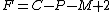# Phase Rule

Also found in: Dictionary, Medical, Wikipedia.
Related to Phase Rule: Phase diagram

## Phase rule

A relationship used to determine the number of state variables F, usually chosen from among temperature, pressure, and species compositions in each phase, which must be specified to fix the thermodynamic state of a system in equilibrium. It was derived by J. Willard Gibbs. The phase rule (in the absence of electric, magnetic, and gravitational phenomena) is given by the equation below,where C is the number of chemical species present at equilibrium, P is the number of phases, and M is the number of independent chemical reactions. Here phase is used to indicate a homogeneous, mechanically separable portion of the system, and the term independent reactions refers to the smallest number of chemical reactions which, upon forming various linear combinations, includes all reactions which occur among the species present. The number of independent state variables F is referred to as the degrees of freedom or variance of the system. See Thermodynamic processes

## Phase Rule

a relation in thermodynamics, according to which the sum of the number φ of phases and the variance v for any equilibrium system is equal to the sum of the number k of components and the number n of parameters that specify the equilibrium state of the system: φ + v = k + n. The state parameters—such as temperature T, pressure p, or electric and magnetic field strengths—must have the same values in all phases. If the state of a system can change only as a result of changes in T and p and if the dimensions of the phases are such that the surface energies are negligible, the phase rule has the form

v = k + 2 – φ

For condensed systems, such as alloys of metals, where p either is constant or changes so insignificantly that it does not affect the equilibrium state, the phase rule has the form v = k + 1 – φ; if p changes and T is constant, the rule has the same form. If coexisting phases have the same composition, as at the extrema of both composition-vapor pressure diagrams (seeKONOVALOV’S RULES) and composition-crystallization temperature diagrams (seeROOZEBOOM’S RULES), then the system behaves as a unary system, or a system consisting of one component; that is, v = 3 – φ if both p and T are variables, or v = 2 – φ if either p or T is constant. Finally, when a chemical compound is formed in a system, k is equal to the difference between the number of chemically distinct substances and the number of independent reactions.

Examples of the phase rule may be found in such substances as sulfur and such systems as a solution consisting of water and salt. In a single phase (for which v = 1 – 1 + 2 = 2), particularly in the gaseous phase, sulfur is a bivariant system; that is, T and p may change independently of one another over a certain range. In two phases (for which v = 1 – 2 + 2 = 1), for example, as a boiling liquid in equilibrium with a vapor, sulfur is a univariant system; that is, only T or p may change, since the two parameters are functions of one another (seeCLAPEYRON EQUATION). In three phases (for which v = 1 – 3 + 2 = 0), particularly as rhombic sulfur in equilibrium with the liquid and gaseous phases, sulfur is an invariant system; that is, the three phases coexist at unique values of p and T (seeTRIPLE POINT). A solution consisting of water and salt is a Invariant system if one phase is present or an invariant system if four phases are present. The single phase may be a solution for which T, p, and the concentration c may change over a known range; the four phases—namely, the aqueous solution, salt, ice, and water vapor—can coexist at a unique combination of T, p, and c.

The phase rule was derived by J. Gibbs and published in 1876. It was widely used in the late 19th and early 20th centuries by J. van’t Hoff, H. Roozeboom, and their students, as well as by N. S. Kurnakov and those who employed his methods. The phase rule is of especially great importance in the study of heterogeneous systems, particularly in metal science, metallurgy, petrology, and chemical engineering. (See alsoDIAGRAM, CHEMICAL; STRUCTURAL DIAGRAM; and .)

### REFERENCES

Gibbs, J. W. “O sushchestvuiushchikh fazakh materii.” In his Termodinamicheskie raboty. Moscow-Leningrad, 1950. Pages 143–48. (Translated from English.)
Anosov, V. Ia., and S. A. Pogodin. Osnovnye nachala fiziko-khimicheskogo analiza. Moscow-Leningrad, 1947.
Dreving, V. P., and Ia. A. Kalashnikov. Pravilofaz s izlozheniem osnov termodinamiki [2nd ed.]. [Moscow] 1964.
Storonkin, A. V. Termodinamika geterogennykh sistem, parts 1–3. [Leningrad] 1967–69.
Karapet’iants, M. Kh. Khimicheskaia termodinamika, 3rd ed. Moscow, 1975.

M. KH. KARAPET’IANTS

## phase rule

[′fāz rül]
(physical chemistry)
Site: Follow: Share:
Open / Close